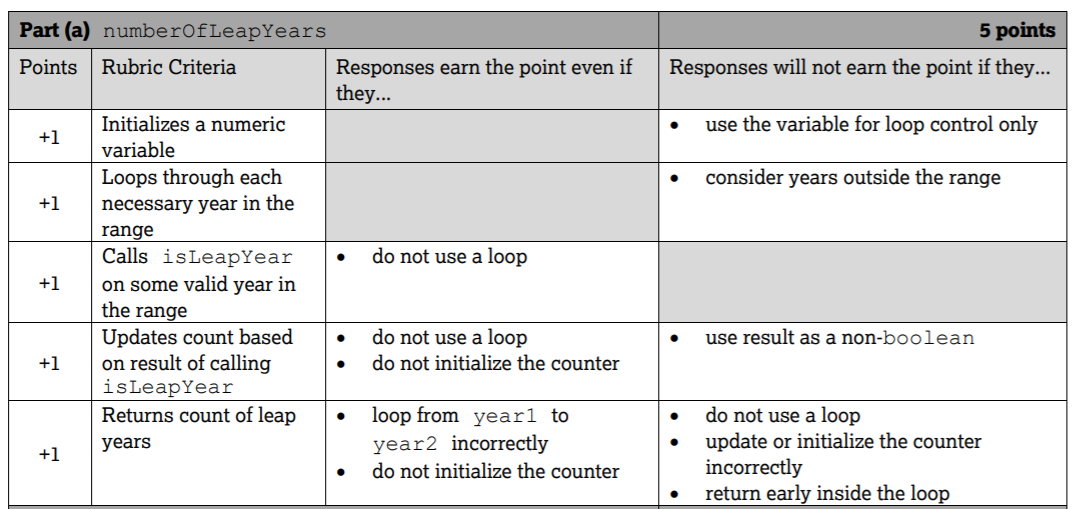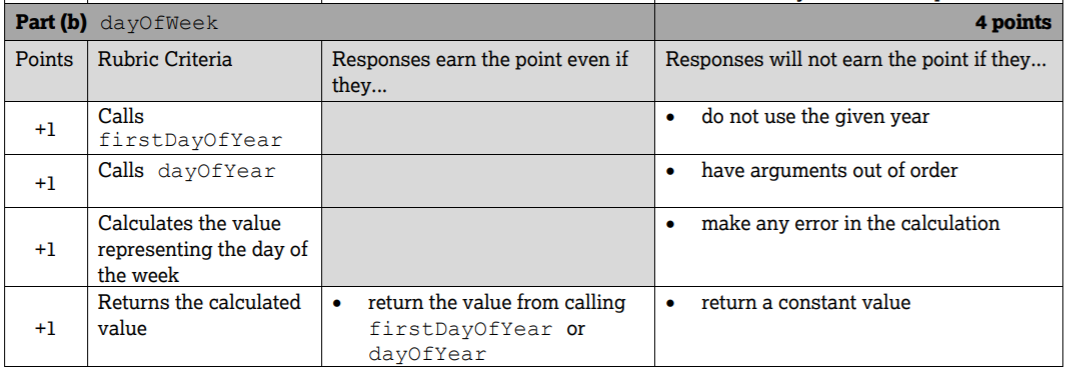# 4.11. Free Response Questions (FRQs) for Control Structures¶

The AP exam’s first free response question (FRQ) is on Methods and Control Structures as of 2019. This question 1 uses expressions, loops, and if statements. The AP exam provides the method header with some parameter variables and other methods that you will need to call in the solution. This question does not involve more complex topics such as arrays.

FRQ Question 1 on Control Structures will probably involve:

• a for-loop that probably uses the method’s parameter variables,

• an if statement, probably inside the loop,

• calls to other class methods given to you,

• a numerical or string value that is calculated by the loop and returned at the end of the method.

• if the question has 2 parts, 1 part will probably require a loop and the other just an expression.

On question 1, you will get points for:

• constructing the loop correctly,

• calling the correct methods,

• calculating the correct value,

• and returning the value.

Try to have some code for each of these steps. Do not use arrays or other more complex code. You may need to use Math or string methods.

## 4.11.1. 2019 APCalendar FRQ¶

The 2019 FRQ 1 is a good example of what to expect. It is available as question 1 on pages 3-6 of https://apstudents.collegeboard.org/sites/default/files/2019-05/ap19-frq-computer-science-a.pdf , reproduced below.

Question 1.

The APCalendar class contains methods used to calculate information about a calendar. You will write two methods of the class.

```public class APCalendar
{
/** Returns true if year is a leap year and false otherwise. */
private static boolean isLeapYear(int year)
{ /* implementation not shown */ }

/** Returns the number of leap years between year1 and year2, inclusive.
* Precondition: 0 <= year1 <= year2
*/
public static int numberOfLeapYears(int year1, int year2)
{ /* to be implemented in part (a) */ }

/** Returns the value representing the day of the week for the first day of year,
* where 0 denotes Sunday, 1 denotes Monday, ..., and 6 denotes Saturday.
*/
private static int firstDayOfYear(int year)
{ /* implementation not shown */ }

/** Returns n, where month, day, and year specify the nth day of the year.
* Returns 1 for January 1 (month = 1, day = 1) of any year.
* Precondition: The date represented by month, day, year is a valid date.
*/
private static int dayOfYear(int month, int day, int year)
{ /* implementation not shown */ }

/** Returns the value representing the day of the week for the given date
* (month, day, year), where 0 denotes Sunday, 1 denotes Monday, ...,
* and 6 denotes Saturday.
* Precondition: The date represented by month, day, year is a valid date.
*/
public static int dayOfWeek(int month, int day, int year)
{ /* to be implemented in part (b) */ }

// There may be instance variables, constructors, and other methods not shown.
}
```

## 4.11.2. Part A: numberOfLeapYear()¶

Write the static method numberOfLeapYears, which returns the number of leap years between year1 and year2, inclusive. In order to calculate this value, a helper method is provided for you.

• isLeapYear(year) returns true if year is a leap year and false otherwise.

Complete method numberOfLeapYears below. You must use isLeapYear appropriately to receive full credit.

```/** Returns the number of leap years between year1 and year2, inclusive.
* Precondition: 0 <= year1 <= year2
*/
public static int numberOfLeapYears(int year1, int year2)
```

## 4.11.3. How to solve numberOfLeapYears()¶

First, circle the information given that you will need to use:

• the parameters year1 and year2

• the isLeapYear(year) method

Also, circle what the return type of what you need to return. In this case, the return type of numberOfLeapYears is int and you need to calculate the number of leap years between year1 and year2 and return it. Declare a variable for this return value and return it at the end of the method to get 1 point.

```/** Returns the number of leap years between year1 and year2, inclusive.
* Precondition: 0 <= year1 <= year2
*/
public static int numberOfLeapYears(int year1, int year2)
{
int numLeapYears = 0;
// Your loop will go in here

return numLeapYears;
}
```

Next, plan your loop. Click to reveal some problems that may help you to plan the loop.

Write the code for the method numberOfLeapYears below and run to test it.

In the 2019 AP exam, part A numberOfLeapYears method was worth 5 points using the rubric below. Did you receive all 5 points? In class, your teacher may have you grade each others’ code.Figure 1: Rubric for the numberOfLeapYears method¶

## 4.11.4. Part B: dayOfWeek()¶

In part B of the AP Calendar FRQ, you need to write the code inside a static method dayOfWeek, which returns the integer value representing the day of the week for the given date (month, day, year), where 0 denotes Sunday, 1 denotes Monday, …, and 6 denotes Saturday. This seems difficult at first, but helper methods are given to you to do most of the work. You just need to put them together to calculate the value. The helper methods given to you are:

• firstDayOfYear(year) returns the integer value representing the day of the week for the first day of year, where 0 denotes Sunday, 1 denotes Monday, …, and 6 denotes Saturday. For example, since 2019 began on a Tuesday, firstDayOfYear(2019) returns 2.

• dayOfYear(month, day, year) returns n, where month, day, and year specify the nth day of the year. For the first day of the year, January 1 (month = 1, day = 1), the value 1 is returned. This method accounts for whether year is a leap year. For example, dayOfYear(3, 1, 2017) returns 60, since 2017 is not a leap year, while dayOfYear(3, 1, 2016) returns 61, since 2016 is a leap year.

If you know that 1/1/2019 was a Tuesday (2) using the firstDayYear method, and you know that today is the nth day of the year using the dayOfYear method, you can figure out what day of the week today is by adding those together. Try some examples by revealing the problems below.

The FRQ that involves writing an expression will probably use the mod % operator. Remember these tips about when to use the mod operator:

• Use mod whenever you need to wrap around to the front if the value goes over the limit (num % limit). For example here for weekdays or for hours and minutes.

• Use mod to check for odd or even numbers (num % 2 == 1) is odd and (num % 2 == 0) is even. Actually, you can use it to check if any number is evenly divisible by another (num1 % num2 == 0)

• Use mod to get the last digit from an integer number (num % 10 = last digit on right).

Try the mod operator below.

Complete the code for the method dayOfWeek below for Part B of this FRQ.

Write the code for the method dayOfWeek below and run to test it. Then, try it with today’s date and see if it returns the right value.

In the 2019 AP exam, part B dayOfWeek method was worth 4 points using the rubric below. Did you receive all 4 points? In class, your teacher may have you grade each others’ code.Figure 2: Rubric for the dayOfWeek method¶
You have attempted of activities on this page